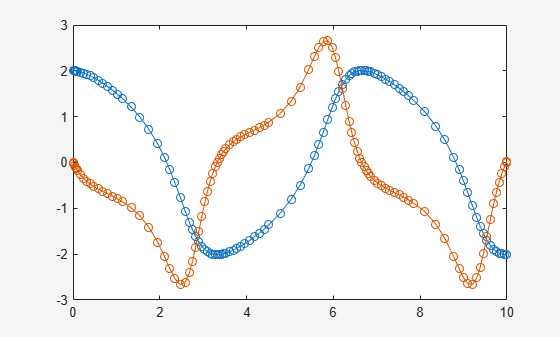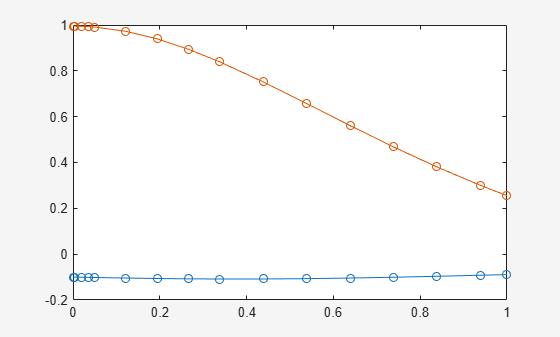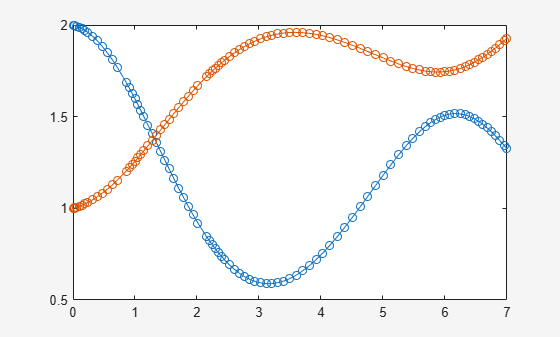odeFunction

Convert symbolic expressions to function handle for ODE solvers

Description

example

f = odeFunction(expr,vars) converts a system of symbolic algebraic expressions to a MATLAB® function handle. This function handle can be used as input to the numerical MATLAB ODE solvers, except for ode15i. The argument vars specifies the state variables of the system.

example

f = odeFunction(expr,vars,p1,...,pN) specifies the symbolic parameters of the system as p1,...,pN.

example

f = odeFunction(___,Name,Value) uses additional options specified by one or more Name,Value pair arguments.

Examples

collapse all

Convert a system of symbolic differential algebraic equations to a function handle suitable for the MATLAB ODE solvers. Then solve the system by using the ode15s solver.

Create the following second-order differential algebraic equation.

syms y(t);
eqn = diff(y(t),t,2) == (1-y(t)^2)*diff(y(t),t) - y(t);

Use reduceDifferentialOrder to rewrite that equation as a system of two first-order differential equations. Here, vars is a vector of state variables of the system. The new variable Dy(t) represents the first derivative of y(t) with respect to t.

[eqs,vars] = reduceDifferentialOrder(eqn,y(t))
eqs =
diff(Dyt(t), t) + y(t) + Dyt(t)*(y(t)^2 - 1)
Dyt(t) - diff(y(t), t)

vars =
y(t)
Dyt(t)

Set initial conditions for y(t) and its derivative Dy(t) to 2 and 0 respectively.

initConditions = [2 0];

Find the mass matrix M of the system and the right sides of the equations F.

[M,F] = massMatrixForm(eqs,vars)
M =
[  0, 1]
[ -1, 0]

F =
- y(t) - Dyt(t)*(y(t)^2 - 1)
-Dyt(t)

M and F refer to the form $M\left(t,x\left(t\right)\right)\stackrel{˙}{x}\left(t\right)=F\left(t,x\left(t\right)\right).$. To simplify further computations, rewrite the system in the form $\stackrel{˙}{x}\left(t\right)=f\left(t,x\left(t\right)\right)$.

f = M\F
f =
Dyt(t)
- Dyt(t)*y(t)^2 - y(t) + Dyt(t)

Convert f to a MATLAB function handle by using odeFunction. The resulting function handle is input to the MATLAB ODE solver ode15s.

odefun = odeFunction(f,vars);
ode15s(odefun, [0 10], initConditions)Convert a system of symbolic differential equations containing both state variables and symbolic parameters to a function handle suitable for the MATLAB ODE solvers.

Create the system of differential algebraic equations. Here, the symbolic functions x1(t) and x2(t) represent the state variables of the system. The system also contains constant symbolic parameters a, b, and the parameter function r(t). These parameters do not represent state variables. Specify the equations and state variables as two symbolic vectors: equations as a vector of symbolic equations, and variables as a vector of symbolic function calls.

syms x1(t) x2(t) a b r(t)
eqs = [diff(x1(t),t) == a*x1(t) + b*x2(t)^2,...
x1(t)^2 + x2(t)^2 == r(t)^2];
vars = [x1(t) x2(t)];

Find the mass matrix M and vector of the right side F for this system. M and F refer to the form $M\left(t,x\left(t\right)\right)\stackrel{˙}{x}\left(t\right)=F\left(t,x\left(t\right)\right).$.

[M,F] = massMatrixForm(eqs,vars)
M =
[ 1, 0]
[ 0, 0]

F =
b*x2(t)^2 + a*x1(t)
r(t)^2 - x1(t)^2 - x2(t)^2

Use odeFunction to generate MATLAB function handles from M and F. The function handle F contains symbolic parameters.

M = odeFunction(M,vars)
F = odeFunction(F,vars,a,b,r(t))
M =
function_handle with value:
@(t,in2)reshape([1.0,0.0,0.0,0.0],[2,2])

F =
function_handle with value:
@(t,in2,param1,param2,param3)[param1.*in2(1,:)+...
param2.*in2(2,:).^2;param3.^2-in2(1,:).^2-in2(2,:).^2]

Specify the parameter values.

a = -0.6;
b = -0.1;
r = @(t) cos(t)/(1+t^2);

Create the reduced function handle F.

F = @(t,Y) F(t,Y,a,b,r(t));

Specify consistent initial conditions for the DAE system.

t0 = 0;
y0 = [-r(t0)*sin(0.1); r(t0)*cos(0.1)];
yp0 = [a*y0(1) + b*y0(2)^2; 1.234];

Create an option set that contains the mass matrix M of the system and vector yp0 of initial conditions for the derivatives.

opt = odeset('mass',M,'InitialSlope',yp0);

Now, use ode15s to solve the system of equations.

ode15s(F, [t0, 1], y0, opt)Write the generated function handles to files by using the File option. When writing to files, odeFunction optimizes the code using intermediate variables named t0, t1, .… Include comments the files by specifying the Comments option.

Define the system of differential equations. Find the mass matrix M and the right side F.

syms x(t) y(t)
eqs = [diff(x(t),t)+2*diff(y(t),t) == 0.1*y(t), ...
x(t)-y(t) == cos(t)-0.2*t*sin(x(t))];
vars = [x(t) y(t)];
[M,F] = massMatrixForm(eqs,vars);

Write the MATLAB code for M and F to the files myfileM and myfileF. odeFunction overwrites existing files. Include the comment Version: 1.1 in the files You can open and edit the output files.

function expr = myfileM(t,in2)
%MYFILEM
%    EXPR = MYFILEM(T,IN2)

%    This function was generated by the Symbolic Math Toolbox version 7.3.
%    01-Jan-2017 00:00:00

%Version: 1.1
expr = reshape([1.0,0.0,2.0,0.0],[2, 2]);
function expr = myfileF(t,in2)
%MYFILEF
%    EXPR = MYFILEF(T,IN2)

%    This function was generated by the Symbolic Math Toolbox version 7.3.
%    01-Jan-2017 00:00:00

%Version: 1.1
x = in2(1,:);
y = in2(2,:);
expr = [y.*(1.0./1.0e1);-x+y+cos(t)-t.*sin(x).*(1.0./5.0)];

Specify consistent initial values for x(t) and y(t) and their first derivatives.

xy0 = [2; 1];    % x(t) and y(t)
xyp0 = [0; 0.05*xy0(2)];    % derivatives of x(t) and y(t)

Create an option set that contains the mass matrix M, initial conditions xyp0, and numerical tolerances for the numerical search.

opt = odeset('mass', M, 'RelTol', 10^(-6),...
'AbsTol', 10^(-6), 'InitialSlope', xyp0);

Solve the system of equations by using ode15s.

ode15s(F, [0 7], xy0, opt)Use the name-value pair argument 'Sparse',true when converting sparse symbolic matrices to MATLAB function handles.

Create the system of differential algebraic equations. Here, the symbolic functions x1(t) and x2(t) represent the state variables of the system. Specify the equations and state variables as two symbolic vectors: equations as a vector of symbolic equations, and variables as a vector of symbolic function calls.

syms x1(t) x2(t)

a = -0.6;
b = -0.1;
r = @(t) cos(t)/(1 + t^2);

eqs = [diff(x1(t),t) == a*x1(t) + b*x2(t)^2,...
x1(t)^2 + x2(t)^2 == r(t)^2];
vars = [x1(t) x2(t)];

Find the mass matrix M and vector of the right side F for this system. M and F refer to the form $M\left(t,x\left(t\right)\right)\stackrel{˙}{x}\left(t\right)=F\left(t,x\left(t\right)\right).$.

[M,F] = massMatrixForm(eqs,vars)
M =
[ 1, 0]
[ 0, 0]

F =
- (3*x1(t))/5 - x2(t)^2/10
cos(t)^2/(t^2 + 1)^2 - x1(t)^2 - x2(t)^2

Generate MATLAB function handles from M and F. Because most of the elements of the mass matrix M are zeros, use the Sparse argument when converting M.

M = odeFunction(M,vars,'Sparse',true)
F = odeFunction(F,vars)
M =
function_handle with value:
@(t,in2)sparse(,,[1.0],2,2)

F =
function_handle with value:
@(t,in2)[in2(1,:).*(-3.0./5.0)-in2(2,:).^2./1.0e+1;...
cos(t).^2.*1.0./(t.^2+1.0).^2-in2(1,:).^2-in2(2,:).^2]

Specify consistent initial conditions for the DAE system.

t0 = 0;
y0 = [-r(t0)*sin(0.1); r(t0)*cos(0.1)];
yp0= [a*y0(1) + b*y0(2)^2; 1.234];

Create an option set that contains the mass matrix M of the system and vector yp0 of initial conditions for the derivatives.

opt = odeset('mass',M,'InitialSlope', yp0);

Solve the system of equations using ode15s.

ode15s(F, [t0, 1], y0, opt)Input Arguments

collapse all

System of algebraic expressions, specified as a vector of symbolic expressions.

State variables, specified as a vector of symbolic functions or function calls, such as x(t).

Example: [x(t),y(t)] or [x(t);y(t)]

Parameters of the system, specified as symbolic variables, functions, or function calls, such as f(t). You can also specify parameters of the system as a vector or matrix of symbolic variables, functions, or function calls. If expr contains symbolic parameters other than the variables specified in vars, you must specify these additional parameters as p1,...,pN.

Name-Value Arguments

Specify optional comma-separated pairs of Name,Value arguments. Name is the argument name and Value is the corresponding value. Name must appear inside quotes. You can specify several name and value pair arguments in any order as Name1,Value1,...,NameN,ValueN.

Example: odeFunction(expr,vars,'File','myfile')

Comments to include in the file header, specified as a character vector, cell array of character vectors, or string vector.

Path to the file containing generated code, specified as a character vector. The generated file accepts arguments of type double, and can be used without Symbolic Math Toolbox™. If the value is empty, odeFunction generates an anonymous function. If the character vector does not end in .m, the function appends .m.

By default, odeFunction with the File argument generates a file containing optimized code. Optimized means intermediate variables are automatically generated to simplify or speed up the code. MATLAB generates intermediate variables as a lowercase letter t followed by an automatically generated number, for example t32. To disable code optimization, use the Optimize argument.

Flag preventing optimization of code written to a function file, specified as false or true.

By default, odeFunction with the File argument generates a file containing optimized code. Optimized means intermediate variables are automatically generated to simplify or speed up the code. MATLAB generates intermediate variables as a lowercase letter t followed by an automatically generated number, for example t32.

odeFunction without the File argument (or with a file path specified by an empty character vector) creates a function handle. In this case, the code is not optimized. If you try to enforce code optimization by setting Optimize to true, then odeFunction throws an error.

Flag that switches between sparse and dense matrix generation, specified as true or false. When you specify 'Sparse',true, the generated function represents symbolic matrices by sparse numeric matrices. Use 'Sparse',true when you convert symbolic matrices containing many zero elements. Often, operations on sparse matrices are more efficient than the same operations on dense matrices. See Sparse Matrices.

Output Arguments

collapse all

Function handle that can serve as input argument to all numerical MATLAB ODE solvers, except for ode15i, returned as a MATLAB function handle.

odeFunction returns a function handle suitable for the ODE solvers such as ode45, ode15s, ode23t, and others. The only ODE solver that does not accept this function handle is the solver for fully implicit differential equations, ode15i. To convert the system of equations to a function handle suitable for ode15i, use daeFunction.